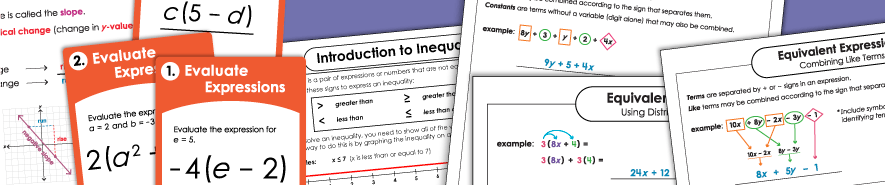# Middle School Math ContentAbsolute Value

These worksheets and printable activities will help students to learn about the absolute value of numbers. Includes identifying values, opposite numbers, ordering, and comparing.

Algebra and Pre-Algebra

Work with basic algebraic expressions, equations, and inequalities. Learn about dependent and independent variables, calculate slope, and more.

Angles: Complementary and Supplementary

This collection contains math resources on supplementary and complementary angles.

Angle Types & Measurement

Learn about acute, obtuse, and right angles. Use a protractor to measure angles.

Area of Circles

Given the radius or diameter, find the areas of the circles.

Areas of Parallelograms

With these task cards and worksheets, your pupils can practice calculating the areas of parallelogram shapes.

Areas of Trapezoids

Area of Triangles

Use the formula A=.5bh to calculate the area of the triangles.

Box Plots (Box-and-Whisker Diagrams)

Create 5-digit summaries with Q1, median, and Q3, minimum, and maximum. Then, use the 5-digit summaries to create box-and-whisker plots.

Dividing Fractions and Mixed Numbers

Practice dividing pairs of fractions and mixed numbers.

Inequalities: Single Variable

This is the most basic level of inequality worksheet. It includes a variable and a number separated by a "greater than" or "less than" sign.
(example: a > 120)

Inequalities: One Step

(example: y + 7 > 15)

Integers (Positive and Negative)

Add, subtract, multiply, and divide positive and negative integers.

Linear Equations

Graph linear equations, find y-intercepts, solve systems of linear equations, and more.

Multiplying Fractions & Mixed Numbers

Practice multiplying fractions and mixed numbers with these printables.

Pythagorean Theorem

Find the lengths of the sides of the right triangles using the Pythagorean theorem formula.

Slope

Calculate slopes using ordered pairs, graphs, and x/y tables.

Surface Area

Find the surface areas of the solid, three-dimensional shapes.

Unit Rate

Calculate unit rates and ratios. Solve unit rate word problems involving pricing, acceleration, and linear measurement.

Volume

Calculate the volumes of cylinders, cones, spheres, and other shapes with these printables.

More Math Worksheets